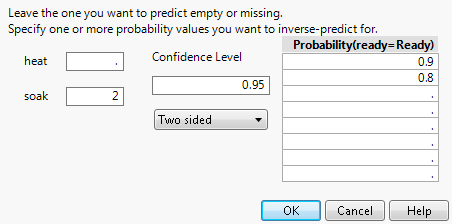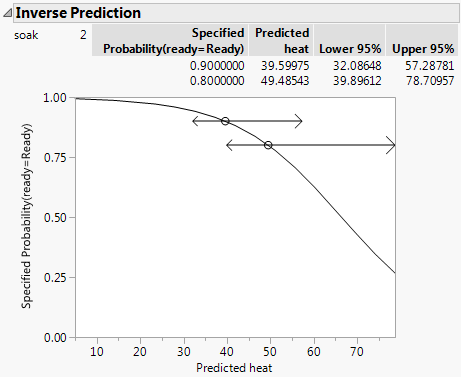Publication date: 11/10/2021

## Example of Inverse Prediction

Inverse prediction enables you to predict the value for one independent variable at a specified probability of the response. If there is more than one independent variable, you must specify values for all of them except the one you are predicting.

An experiment was performed on metal ingots. The ingots were prepared with different heating and soaking times and then tested for readiness to roll. See Cox and Snell (1989). In JMP, the data are contained in the Ingots.jmp sample data table.

You are interested in predicting the heating time at a soaking time of 2 for probabilities of readiness to roll of 0.8 and 0.9.

1. For the analysis, follow the steps in Example of Nominal Logistic Regression.

2. Click the red triangle next to Nominal Logistic Fit for ready and select Inverse Prediction.

3. Delete the value for heat.

You want to find the predicted value of heat, so you leave the heat value empty.

4. Enter 2 for soak.

You want to predict heating time when soak is 2.

5. Under Probability, enter 0.9 and 0.8 in the first two rows.

Figure 11.7 The Inverse Prediction Specification Window6. Click OK.

Figure 11.8 Inverse Prediction ReportWhen soak is 2, the predicted value of heat for which there is a 90% chance of an ingot being ready to roll is 39.60 with a 95% confidence interval from 32.09 to 57.29. When soak is 2, the predicted value for heat for which there is an 80% chance of an ingot being ready to roll is 49.49 with a 95% confidence interval from 39.90 to 78.71.